xArithmetic meanOverview

In mathematics
Mathematics
Mathematics is the study of quantity, space, structure, and change. Mathematicians seek out patterns and formulate new conjectures. Mathematicians resolve the truth or falsity of conjectures by mathematical proofs, which are arguments sufficient to convince other mathematicians of their validity...

and statistics
Statistics
Statistics is the study of the collection, organization, analysis, and interpretation of data. It deals with all aspects of this, including the planning of data collection in terms of the design of surveys and experiments....

, the arithmetic mean, often referred to as simply the mean or average when the context is clear, is a method to derive the central tendency of a sample space. The term "arithmetic mean" is preferred in mathematics and statistics because it helps distinguish it from other mean
Average
In mathematics, an average, or central tendency of a data set is a measure of the "middle" value of the data set. Average is one form of central tendency. Not all central tendencies should be considered definitions of average....

s such as the geometric
Geometric mean
The geometric mean, in mathematics, is a type of mean or average, which indicates the central tendency or typical value of a set of numbers. It is similar to the arithmetic mean, except that the numbers are multiplied and then the nth root of the resulting product is taken.For instance, the...

and harmonic mean
Harmonic mean
In mathematics, the harmonic mean is one of several kinds of average. Typically, it is appropriate for situations when the average of rates is desired....

.

In addition to mathematics and statistics, the arithmetic mean is used frequently in fields such as economics
Economics
Economics is the social science that analyzes the production, distribution, and consumption of goods and services. The term economics comes from the Ancient Greek from + , hence "rules of the house"...

, sociology
Sociology
Sociology is the study of society. It is a social science—a term with which it is sometimes synonymous—which uses various methods of empirical investigation and critical analysis to develop a body of knowledge about human social activity...

, and history
History
History is the discovery, collection, organization, and presentation of information about past events. History can also mean the period of time after writing was invented. Scholars who write about history are called historians...

, though it is used in almost every academic field to some extent.Unanswered QuestionsOf odd number of terms ,middle terms is equal to half of the sum of the first and the last termEncyclopedia
In mathematics
Mathematics
Mathematics is the study of quantity, space, structure, and change. Mathematicians seek out patterns and formulate new conjectures. Mathematicians resolve the truth or falsity of conjectures by mathematical proofs, which are arguments sufficient to convince other mathematicians of their validity...

and statistics
Statistics
Statistics is the study of the collection, organization, analysis, and interpretation of data. It deals with all aspects of this, including the planning of data collection in terms of the design of surveys and experiments....

, the arithmetic mean, often referred to as simply the mean or average when the context is clear, is a method to derive the central tendency of a sample space. The term "arithmetic mean" is preferred in mathematics and statistics because it helps distinguish it from other mean
Average
In mathematics, an average, or central tendency of a data set is a measure of the "middle" value of the data set. Average is one form of central tendency. Not all central tendencies should be considered definitions of average....

s such as the geometric
Geometric mean
The geometric mean, in mathematics, is a type of mean or average, which indicates the central tendency or typical value of a set of numbers. It is similar to the arithmetic mean, except that the numbers are multiplied and then the nth root of the resulting product is taken.For instance, the...

and harmonic mean
Harmonic mean
In mathematics, the harmonic mean is one of several kinds of average. Typically, it is appropriate for situations when the average of rates is desired....

.

In addition to mathematics and statistics, the arithmetic mean is used frequently in fields such as economics
Economics
Economics is the social science that analyzes the production, distribution, and consumption of goods and services. The term economics comes from the Ancient Greek from + , hence "rules of the house"...

, sociology
Sociology
Sociology is the study of society. It is a social science—a term with which it is sometimes synonymous—which uses various methods of empirical investigation and critical analysis to develop a body of knowledge about human social activity...

, and history
History
History is the discovery, collection, organization, and presentation of information about past events. History can also mean the period of time after writing was invented. Scholars who write about history are called historians...

, though it is used in almost every academic field to some extent. For example, per capita GDP gives an approximation of the arithmetic average income of a nation's population.

While the arithmetic mean is often used to report central tendencies
Central tendency
In statistics, the term central tendency relates to the way in which quantitative data is clustered around some value. A measure of central tendency is a way of specifying - central value...

, it is not a robust statistic, meaning that it is greatly influenced by outlier
Outlier
In statistics, an outlier is an observation that is numerically distant from the rest of the data. Grubbs defined an outlier as: An outlying observation, or outlier, is one that appears to deviate markedly from other members of the sample in which it occurs....

s. Notably, for skewed distributions, the arithmetic mean may not accord with one's notion of "middle", and robust statistics such as the median
Median
In probability theory and statistics, a median is described as the numerical value separating the higher half of a sample, a population, or a probability distribution, from the lower half. The median of a finite list of numbers can be found by arranging all the observations from lowest value to...

may be a better description of central tendency.

## Definition

Suppose we have sample space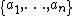. Then the arithmetic mean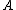is defined via the equation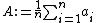.

If the list is a statistical population
Statistical population
A statistical population is a set of entities concerning which statistical inferences are to be drawn, often based on a random sample taken from the population. For example, if we were interested in generalizations about crows, then we would describe the set of crows that is of interest...

, then the mean of that population is called a population mean. If the list is a statistical sample
Sampling (statistics)
In statistics and survey methodology, sampling is concerned with the selection of a subset of individuals from within a population to estimate characteristics of the whole population....

, we call the resulting statistic
Statistic
A statistic is a single measure of some attribute of a sample . It is calculated by applying a function to the values of the items comprising the sample which are known together as a set of data.More formally, statistical theory defines a statistic as a function of a sample where the function...

a sample mean.

## Motivating properties

The arithmetic mean has several properties that make it useful, especially as a measure of central tendency. These include:
• If numbers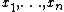have mean X, then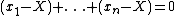. Since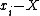is the distance from a given number to the mean, one way to interpret this property is as saying that the numbers to the left of the mean are balanced by the numbers to the right of the mean. The mean is the only single number for which the residuals defined this way sum to zero.
• If it is required to use a single number X as an estimate for the value of numbers, then the arithmetic mean does this best, in the sense of minimizing the sum of squares (xi − X)2 of the residuals
Errors and residuals in statistics
In statistics and optimization, statistical errors and residuals are two closely related and easily confused measures of the deviation of a sample from its "theoretical value"...

. (It follows that the mean is also the best single predictor in the sense of having the lowest root mean squared error.)
• For a normal distribution, the arithmetic mean is equal to both the median and the mode, other measures of central tendency.

## Problems

The arithmetic mean may be misinterpreted as the median to imply that most values are higher or lower than is actually the case. If elements in the sample space increase arithmetically
Arithmetic progression
In mathematics, an arithmetic progression or arithmetic sequence is a sequence of numbers such that the difference between the consecutive terms is constant...

, when placed in some order, then the median and arithmetic average are equal. For example, consider the sample space {1,2,3,4}. The average is 2.5, as is the median. However, when we consider a sample space that cannot be arranged into an arithmetic progression, such as {1,2,4,8,16}, the median and arithmetic average can differ significantly. In this case the arithmetic average is 6.2 and the median is 4. When one looks at the arithmetic average of a sample space, one must note that the average value can vary significantly from most values in the sample space.

There are applications of this phenomenon in many fields. For example, since the 1980s in the United States median income has increased more slowly than the arithmetic average of income. Researchers dealing with frequency data must also be careful when reporting summary statistics such as means or median. Where a phenomenon is rare in general (for example, emergency room visits among the general population), but occurs frequently in some people (for example, dare devils), then the mean value may be much lower than the median.

## Angles

Particular care must be taken when using cyclic data such as phases or angle
Angle
In geometry, an angle is the figure formed by two rays sharing a common endpoint, called the vertex of the angle.Angles are usually presumed to be in a Euclidean plane with the circle taken for standard with regard to direction. In fact, an angle is frequently viewed as a measure of an circular arc...

s. Naïvely taking the arithmetic mean of 1° and 359° yields a result of 180°.
This is incorrect for two reasons:
• Firstly, angle measurements are only defined up to a factor of 360°
Degree (angle)
A degree , usually denoted by ° , is a measurement of plane angle, representing 1⁄360 of a full rotation; one degree is equivalent to π/180 radians...

(or 2π, if measuring in radian
Radian
Radian is the ratio between the length of an arc and its radius. The radian is the standard unit of angular measure, used in many areas of mathematics. The unit was formerly a SI supplementary unit, but this category was abolished in 1995 and the radian is now considered a SI derived unit...

s). Thus one could as easily call these 1° and −1°, or 1° and 719° – each of which gives a different average.
• Secondly, in this situation, 0° (equivalently, 360°) is geometrically a better average value: there is lower dispersion
Statistical dispersion
In statistics, statistical dispersion is variability or spread in a variable or a probability distribution...

about it (the points are both 1° from it, and 179° from 180°, the putative average).

In general application such an oversight will lead to the average value artificially moving towards the middle of the numerical range. A solution to this problem is to use the optimization formulation (viz, define the mean as the central point: the point about which one has the lowest dispersion), and redefine the difference as a modular distance (i.e., the distance on the circle: so the modular distance between 1° and 359° is 2°, not 358°).

## See also

• Assumed mean
Assumed mean
In statistics the assumed mean is a method for calculating the arithmetic mean and standard deviation of a data set. It simplifies calculating accurate values by hand. Its interest today is chiefly historical but it can be used to quickly estimate these statistics...

• Average
Average
In mathematics, an average, or central tendency of a data set is a measure of the "middle" value of the data set. Average is one form of central tendency. Not all central tendencies should be considered definitions of average....

• Central tendency
Central tendency
In statistics, the term central tendency relates to the way in which quantitative data is clustered around some value. A measure of central tendency is a way of specifying - central value...

• Consensus forecasts
Consensus forecasts
In a number of sciences, ranging from econometrics to meteorology, consensus forecasts are predictions of the future that are created by combining together several separate forecasts which have often been created using different methodologies...

• Empirical measure
Empirical measure
In probability theory, an empirical measure is a random measure arising from a particular realization of a sequence of random variables. The precise definition is found below. Empirical measures are relevant to mathematical statistics....

• Fréchet mean
Fréchet mean
The Fréchet mean , is the point, x, that minimizes the Fréchet function, in cases where such a unique minimizer exists. The value at a point p, of the Fréchet function associated to a random point X on a complete metric space is the expected squared distance from p to X...

• Generalized mean
Generalized mean
In mathematics, a generalized mean, also known as power mean or Hölder mean , is an abstraction of the Pythagorean means including arithmetic, geometric, and harmonic means.-Definition:...

• Geometric mean
Geometric mean
The geometric mean, in mathematics, is a type of mean or average, which indicates the central tendency or typical value of a set of numbers. It is similar to the arithmetic mean, except that the numbers are multiplied and then the nth root of the resulting product is taken.For instance, the...

• Harmonic mean
Harmonic mean
In mathematics, the harmonic mean is one of several kinds of average. Typically, it is appropriate for situations when the average of rates is desired....

• Inequality of arithmetic and geometric means
Inequality of arithmetic and geometric means
In mathematics, the inequality of arithmetic and geometric means, or more briefly the AM–GM inequality, states that the arithmetic mean of a list of non-negative real numbers is greater than or equal to the geometric mean of the same list; and further, that the two means are equal if and only if...

• Mean
Mean
In statistics, mean has two related meanings:* the arithmetic mean .* the expected value of a random variable, which is also called the population mean....

• Median
Median
In probability theory and statistics, a median is described as the numerical value separating the higher half of a sample, a population, or a probability distribution, from the lower half. The median of a finite list of numbers can be found by arranging all the observations from lowest value to...

• Mode
Mode (statistics)
In statistics, the mode is the value that occurs most frequently in a data set or a probability distribution. In some fields, notably education, sample data are often called scores, and the sample mode is known as the modal score....

• Muirhead's inequality
Muirhead's inequality
In mathematics, Muirhead's inequality, named after Robert Franklin Muirhead, also known as the "bunching" method, generalizes the inequality of arithmetic and geometric means.-The "a-mean":For any real vectora=...

• Sample mean and covariance
• Sample size
Sample size
Sample size determination is the act of choosing the number of observations to include in a statistical sample. The sample size is an important feature of any empirical study in which the goal is to make inferences about a population from a sample...

• Standard deviation
Standard deviation
Standard deviation is a widely used measure of variability or diversity used in statistics and probability theory. It shows how much variation or "dispersion" there is from the average...

• Summary statistics
Summary statistics
In descriptive statistics, summary statistics are used to summarize a set of observations, in order to communicate the largest amount as simply as possible...

• Variance
Variance
In probability theory and statistics, the variance is a measure of how far a set of numbers is spread out. It is one of several descriptors of a probability distribution, describing how far the numbers lie from the mean . In particular, the variance is one of the moments of a distribution...

## Further reading

• Darrell Huff
Darrell Huff
Darrell Huff was an American writer, and is best known as the author of How to Lie with Statistics , the best-selling statistics book of the second half of the twentieth century....

, How to lie with statistics, Victor Gollancz, 1954 (ISBN 0-393-31072-8).

## External links

The source of this article is wikipedia, the free encyclopedia.  The text of this article is licensed under the GFDL.